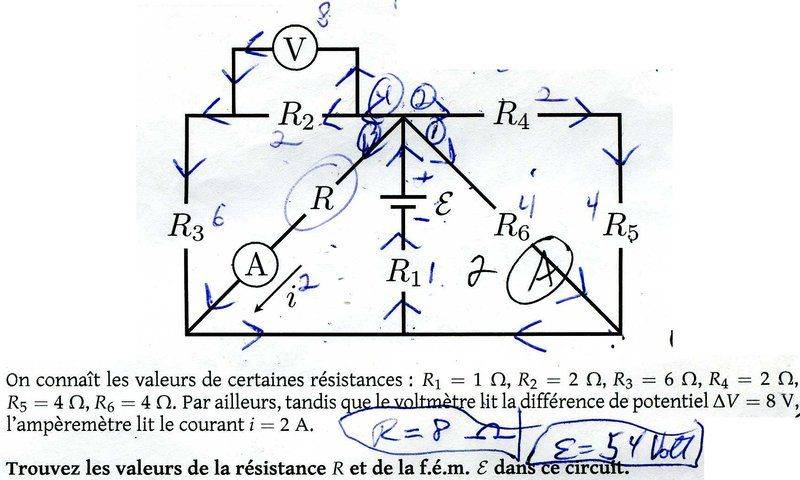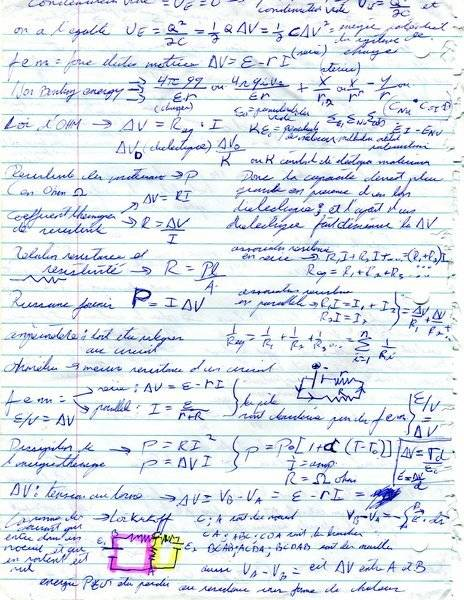# Find the resistance and EMF in a circuit

## Homework Statementtranslation ; we know the values of
R1 = 1 ohm
R2= 2 ohm
R3= 6 ohm
R4= 2 ohm
R5= 4 ohm
R6= 4 ohm

we can also see the voltmeter reading a potential difference value of 8 volts , and the ampere meter giving a reading of 2 amps at the indicated locations

2. Relevant## The Attempt at a Solution

;
[/B]
so , as you can see with the following attachements i ended up with value for the missing resitance of 8 ohm and a f.e.m ( electro force ) oh 54.

by plugging those in we get a potential difference of 8V and a 2 amp signal .

but i got those value by testing a real circuit, and cant get the same numbers through formulas. by symetry , shouldnt the right side have the same potential difference of 8 volts as the left side ?
pretty sure my resistance value of 8 ohm looks ok .

am i wrong in my calculations ? if my handwriting is too bad i can rewrite the equations here . we know the current coming in at a junction has to be the same as the current coming out.
since there are only resistance and only 1 battery , im sure i already used the formula for the answer in one of my worksheet. . i know since i ended up with a value of 8R., gave me a hint that the missing R value was 8 .

#### Attachments

haruspex
Homework Helper
Gold Member
I would find your handwritten working illegible even if I were fluent in French.
Please type in algebra, reserving images for diagrams and textbook extracts.

What is the current through R2?
What is the current through R3?
What is the potential drop across R3?
What is the potential drop across R?

•scottdave and BvU
potential drop and current across a resistance we get from R=delta V / i so
current = delta V / R
potential = R * i

R2 = 2 ohm
R3 = 6 ohm

so V= R2*i ---> 8 = 2*i ----> i = 8/2 = 4

current after R3 should be 2 amps because current after R is 2amps ,
no?

both are in series . but are in parallele with the unkown resistance R so the equivalent resitance for those 3 should be
2 + 6 = 8 ohm , then 1/8 + 1/R = the equivalent resistance value . same as ( 8 * R) / (8 + R ) = right side of circuit equivalent resistance .
so current should be

haruspex
Homework Helper
Gold Member
current after R3 should be 2 amps
Not sure what you mean by "current after R3". I asked about current through R3.
Where does the current through R2 go next?

amp meter reads 2 amps , after the unknow resistance . which is the same junction after R3 .
as you can see from the arrows , initial guess for the current flow , suggest that after R2 , current would go on through R3 , then R1 and then to the battery

R2 and R3 are in series , so their total resistance is 8 Ω .

since they're in parrallele with the unknow resistance , and we know the current at the junction where R3 and the unknown resistance meet,

R2 and R3 are in series . so 8v = 8*i so current after going through R2 and R3 is 1 amp ?

scottdave
Homework Helper
Gold Member
Perhaps you should read up on some circuit theory. I'm on my phone right now, but here's something. https://en.m.wikipedia.org/wiki/Node_(circuits)
It is the mobile version of Wikipedia. For a moment, Think about current as flowing through a pipe. If 4 amps (Coulomb's per second) flows through R2, then the same amount must also be flowing through R3

scottdave
Homework Helper
Gold Member
This type of problem should not need a page of calculations. Did i read correctly: you built the circuit and obtained the measurements?

haruspex
Homework Helper
Gold Member
after R2 , current would go on through R3
Right, but it is nothing to do with any guess you have made. R2 and R 3 are in series, with nothing else connected between them. Therefore all the charge that flows through one must flow through the other.
In post #3 you found the current through R 2. So what is the current through R3?

8=6i so 8/6 = 4/3 , would be the current through R3

haruspex
Homework Helper
Gold Member
8=6i
I have no idea how you arrived at that. I cannot tell you what is wrong with your reasoning if you do not post it.

You do not seem to have understood my post #9. I do not see how to spell it out any more clearly without telling you the answer. Please make another attempt to understand it.

if at R2 , we have
8volts = 2 ohm *i

and i isolate i , then i=4

i = 8 / 2 +6 , so i = 1 amp after R3

where are you going with this, i tought we needed to calculate the equivalent resitance value for the entire circuit , then isolate for the last one , using the total circuit values . .

its way easier to get to total effective resistance then you end up with a value for the total resistances and another resistance variable which we are trying to solve for.
. which is what i did in the worksheets. 2 other threads i posted i had the right answer right from the start , but someone convinced me otherwise and i ended up failing that questions, thus losing 1% of my final grade ..

this assignement right here is worth 15% of my final grade . so i cant afford to painfully take weeks to resolve this , my domain is chemistry, not physics...

haruspex
Homework Helper
Gold Member
if at R2 , we have
8volts = 2 ohm *i

and i isolate i , then i=4
Right so far, that is the current through R2.
i = 8 / 2 +6 , so i = 1 amp after R3
You seem to have a basic misunderstanding about currents. All of the current that flows through R 2 must then flow through R3. It has nowhere else to go. Likewise, there is no other source of current at that end of R3. So R3 also carries 4A.

Can you now answer my next question in post #2: what is the potential drop across R3?

Perhaps you had better first take scottdave's advice in post #7, though, and refresh your understanding of the basics.

Last edited:
haruspex
Homework Helper
Gold Member
2 other threads i posted i had the right answer right from the start , but someone convinced me otherwise and i ended up failing that questions,
Nobody suggested answer D was wrong. Your first explanation for how you chose D was incorrect (there should have been no m). @BvU suggested a sanity check; you used that but concluded D was wrong. BvU challenged your reason for saying D was wrong. That you went ahead and answered A was down to you. Perhaps you misunderstood BvU's response.

Last edited:
haruspex
Homework Helper
Gold Member
And in thread https://www.physicsforums.com/threa...ic-field-and-dot-product.950190/#post-6018480, you did not have the right answer at the start. With forum help you eventually got the right magnitude. It is unfortunate that nobody noticed you still had the wrong sign until too late, but that might have been avoided if you had included the energy equation in the Relevant Equations part of the template.